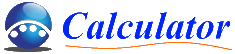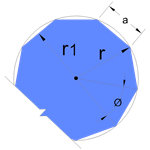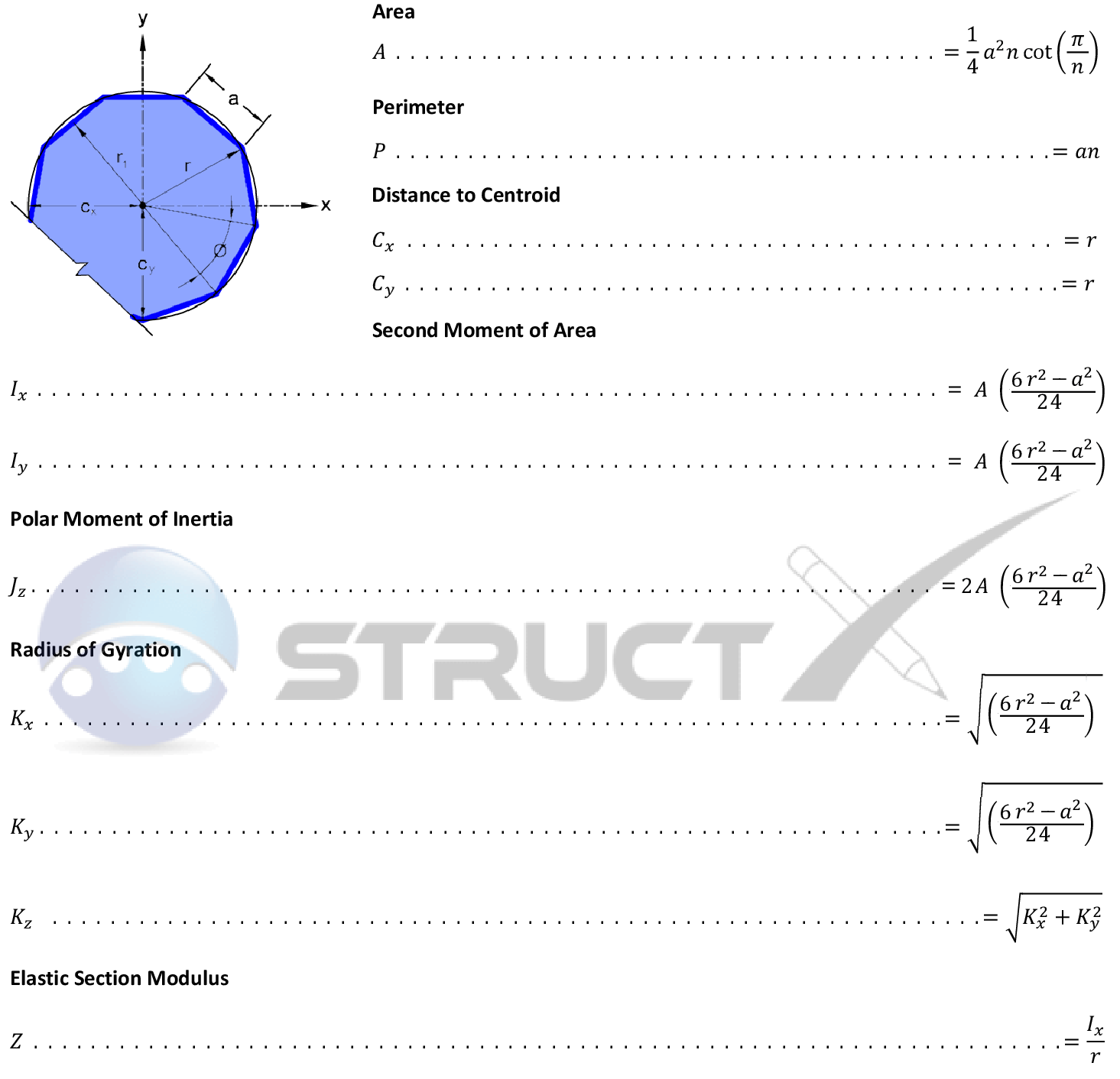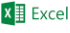# Polygon - Geometric PropertiesMore ShapesGeometric Property CalculatorRadius, r:Total Sides, n: mmmm
 Side, a: mm Area, A: mm2 Perimeter, P: mm Centroid, Cx: mm Centroid, Cy: mm Second Moment of Area, Ix: mm4 Second Moment of Area, Iy: mm4 Polar Moment of Inertia, Jz: mm4 Radius of Gyration, Kx: mm Radius of Gyration, Ky: mm Radius of Gyration, Kz: mm Elastic Section Modulus, Z: mm3

The above polygon property calculator is based on the provided equations and does not account for all mathematical limitations. The calculator has been provided with educational purposes in mind and should be used accordingly. Unit conversionNeed anspreadsheet for designing the above shape, click here!

# Notation and Units

## Metric and Imperial Units

The above formulas may be used with both imperial and metric units. As with all calculations care must be taken to keep consistent units throughout. Examples of units which are typically adopted are outlined below:

# Notation

• A = Geometric Area, in2 or mm2
• a = Length of flat section, in or mm
• C = Distance to Centroid, in or mm
• I = Second moment of area, in4 or mm4
• Ji = Polar Moment of Inertia, in4 or mm4
• K = Radius of Gyration, in or mm
• n = Number of flat sides
• P = Perimeter of shape, in or mm
• r = Outer radius of shape, in or mm
• r1 = radius to flat of shape, in or mm
• Z = Elastic Section Modulus, in3 or mm3

# Online Polygon Property Calculator

Using the structural engineering calculator located at the top of the page (simply click on the the "show/hide calculator" button) the following properties can be calculated:

• Calculate the Area of a Polygon
• Calculate the Perimeter of a Polygon
• Calculate the Centroid of a Polygon
• Calculate the Second Moment of Area (or moment of inertia) of a Polygon
• Calculate the Polar Moment of Inertia of a Polygon
• Calculate the Radius of Gyration of a Polygon
• Calculate the Elastic Section Modulus of a Polygon
• Calculate the Plastic Section Modulus of a Polygon